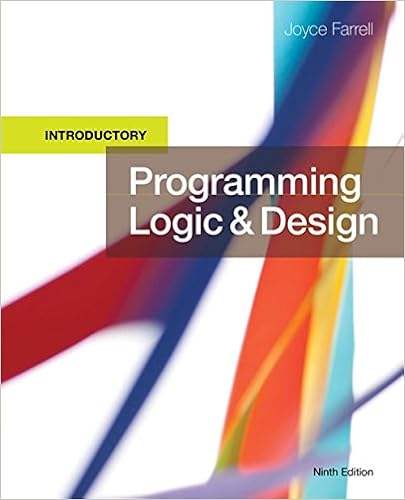# Classnum classnum 1 endwhile return 6 a watson

• Homework Help
• 77
• 98% (249) 243 out of 249 people found this document helpful

This preview shows page 26 - 32 out of 77 pages.

##### We have textbook solutions for you!
The document you are viewing contains questions related to this textbook.The document you are viewing contains questions related to this textbook.
Chapter 6 / Exercise 19
Programming Logic and Design, Introductory
FarrellExpert Verified
classNum = classNum + 1 endwhile return 6. a. Watson Elementary School contains 30 classrooms numbered 1 through 30. Each classroom can contain any number of students up to 35. Each student takes an achievement test at the end of the school year and receives a score from 0 through 100. Write a program that accepts data for each student in the school—student ID, classroom number, and score on the achievement test. Design a program that lists the total points scored for each of the 30 classrooms. 26
##### We have textbook solutions for you!
The document you are viewing contains questions related to this textbook.The document you are viewing contains questions related to this textbook.
Chapter 6 / Exercise 19
Programming Logic and Design, Introductory
FarrellExpert Verified
Programming Logic and Design, 8e Solutions 6- Flowchart: Pseudocode: start Declarations num stuID num classNum num score num SIZE = 30 num QUIT = 9999 num totalPnts[SIZE] = 0 getReady() while stuID <> QUIT collectStudentData() endwhile finishUp() 27
Programming Logic and Design, 8e Solutions 6- stop getReady() output “Enter a student ID or ”, QUIT, “ to quit” input stuID return collectStudentData() output “Enter the classroom number and score for student ”, stuID input classNum, score if classNum >= 1 AND classNum <= SIZE then totalPnts[classNum - 1] = totalPnts[classNum - 1] + score else output “Invalid classroom number” endif output “Enter a student ID or ”, QUIT, “ to quit” input stuID return finishUp() classNum = 0 while classNum < SIZE output classNum + 1, totalPnts[classNum] classNum = classNum + 1 endwhile return b. Modify the Watson Elementary School program so that each classroom’s average of the test scores is output, rather than total scores for each classroom. 28
Programming Logic and Design, 8e Solutions 6- Pseudocode: start Declarations num stuID num classNum num score 29
Programming Logic and Design, 8e Solutions 6- num avg num SIZE = 30 num QUIT = 9999 num totalPnts[SIZE] = 0 num classCnts[SIZE] = 0 getReady() while stuID <> QUIT collectStudentData() endwhile classNum = 0 finishUp() stop getReady() output “Enter a student ID or ”, QUIT, “ to quit” input stuID return collectStudentData() output “Enter the classroom number and score for student ”, stuID input classNum, score if classNum >= 1 AND classNum <= SIZE then totalPnts[classNum - 1] = totalPnts[classNum - 1] + score classCnts[classNum - 1] = classCnts[classNum - 1] + 1 else output “Invalid classroom number” endif output “Enter a student ID or ”, QUIT, “ to quit” input stuID return finishUp() classNum = 0 while classNum < SIZE if classCnts[classNum] = 0 then avg = 0 else avg =totalPnts[classNum]/classCnts[classNum] endif output classNum + 1, avg classNum = classNum + 1 endwhile return 30
Programming Logic and Design, 8e Solutions 6-
•••